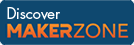Cody

# Problem 23. Finding Perfect Squares

Created by Cody Team in Community

Given a vector of numbers, return true if one of the numbers is a square of one of the other numbers. Otherwise return false.

Example:

``` Input  a = [2 3 4]
Output b is true```

Output is true since 2^2 is 4 and both 2 and 4 appear on the list.

### Solution Stats

39.84% Correct | 60.16% Incorrect
Last solution submitted on Mar 18, 2019

#### TagsMATLAB and Simulink resources for Arduino, LEGO, and Raspberry Pi